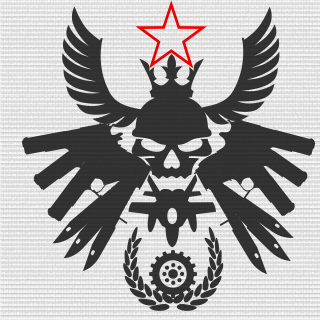-=B.R.A.V.O=-
Tag: [SWAT] Fans: Created: 2013-06-30

Platoon feed

• Nosimelyano joined the platoon -=B.R.A.V.O=-
• MuWKa_Ha_CEPBepe joined the platoon -=B.R.A.V.O=-
• Attackangels joined the platoon -=B.R.A.V.O=-
• maksym34 joined the platoon -=B.R.A.V.O=-
• blastbeat88 joined the platoon -=B.R.A.V.O=-
• MrMadMabizzelGER joined the platoon -=B.R.A.V.O=-
• Fantom942 joined the platoon -=B.R.A.V.O=-
• vlad12523 joined the platoon -=B.R.A.V.O=-
• San9_Pro100_Psix joined the platoon -=B.R.A.V.O=-